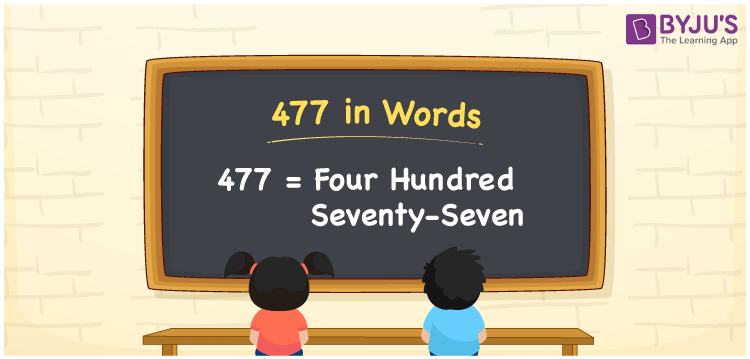# 477 in Words

477 in words is Four hundred seventy-seven. If you bought books for Rs. 477, you can write it as “ I bought books for Rs. Four hundred seventy-seven. The numeral 477 has three digits and it is a natural number. Now, let us have a look at the procedure of writing the number 477 in words using the place value system in detail.

 477 in Words: Four Hundred Seventy-seven. Four Hundred Seventy-seven in Numerical Form: 477.

## 477 in English Words## How to Write 477 in Words?

The numeral 477 has three digits and its place values are given below:

 Hundreds Tens Ones 4 7 7

The expanded form of 477 is as follows:

= 4 × Hundred + 7 × Ten + 7 × One

= 4 × 100 + 7 × 10 + 7 × 1

= 400 + 70 + 7

= 477

= Four hundred seventy-seven

Hence, 477 in words is four hundred seventy-seven.

477 in words – Four hundred seventy-seven

Is 477 an odd number? – Yes

Is 477 an even number? – No

Is 477 a perfect square number? – No

Is 477 a perfect cube number? – No

Is 477 a prime number? – No

Is 477 a composite number? – Yes

## Frequently Asked Questions on 477 in Words

Q1

### Write 477 in words.

477 in words is four hundred seventy-seven.

Q2

### Simplify 400 + 77, and express it in words.

Simplifying 400 + 77, we get 477. Hence, 477 in words is four hundred seventy-seven.

Q3

### Is 477 a prime number?

No, 477 is not a prime number.